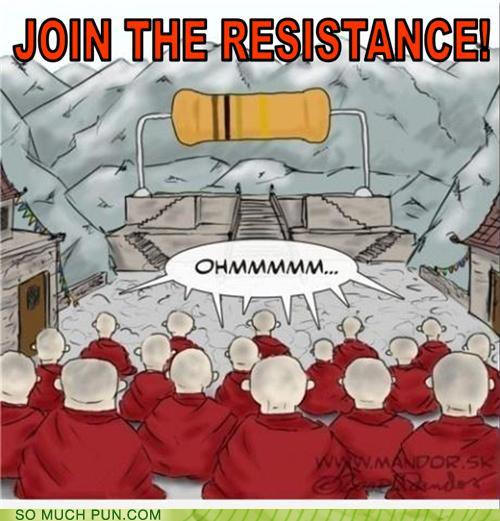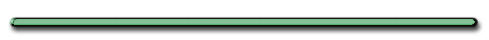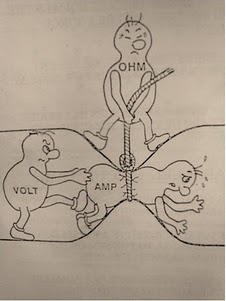Brainiac, Season 1 Episode 4.
Electric Fence Experiment Richard Hammond

School blocks YouTube? Use the file below.1) What is the resistance of .30 m length of copper wire that has a cross-sectional area of 5.0 x 10-5 m2?

1) What is the resistance of .30 m length of copper wire that has a cross-sectional area of 5.0 x 10-5 m2

R = ρL/A

L = .30 m

A = 5.0 x 10-5 m2ρ = 1.72 x 10-8 Ωm

(reference)

 R = 1.72 x 10-8 Ωm(.30 m) 5.0 x 10-5 m2

R = 1.0 x 10-4  Ωa) How could you alter the dimensions of this wire to reduce the resistance?R = ρL/ATo reduce the resistance in a wire you could:

L ?

ShortenA?Increase2) If the length of a wire were halved, how would that change the wires resistance?

R = ρL/A

R, L direct
R would be halvedCross-sectional Area (A) doubled?

R = ρL/A

R, A inverse
R, halved3) Which material on your reference table would produce a wire that would allow current to flow the best?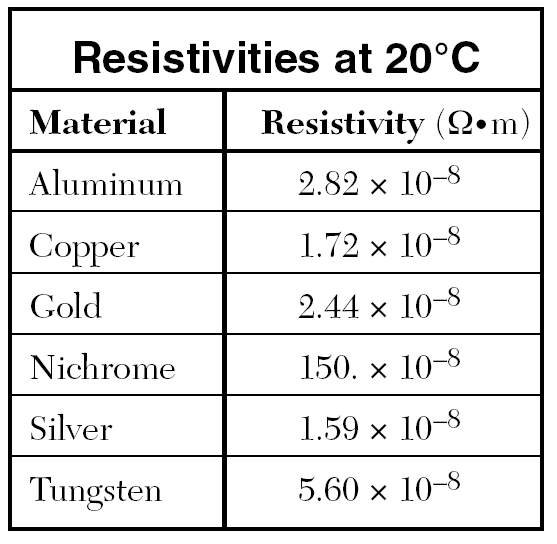Silver, lowest resistivityR, L plot for resistor?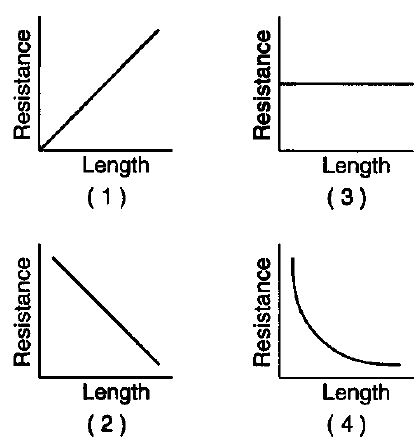(June 1997)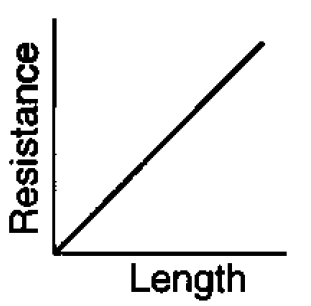Rank the wire resistance from least to greatest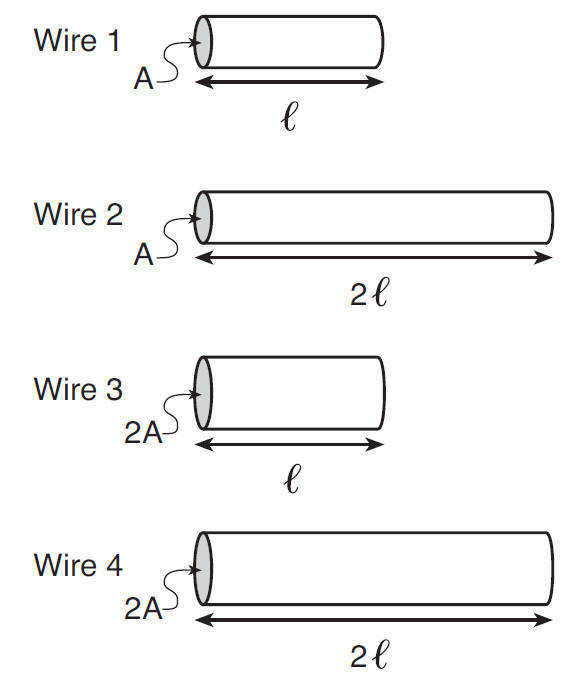June 2017